### 10.5.1.1 Determining a security plan

The notion of a security strategy from Section 9.3.2 extends in a natural way to sequential games. This yields a security plan in which each player performs worst-case analysis by treating the other player as nature under nondeterministic uncertainty. A security plan and its resulting cost can be computed by propagating costs from the leaves up to the root. The computation of the security plan for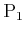for the game in Figure 10.13 is shown in Figure 10.14. The actions that would be chosen by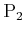are determined at all vertices in the second-to-last level of the tree. Sincetries to maximize costs, the recorded costs at each of these vertices is the maximum over the costs of its children. At the next higher level, the actions that would be chosen byare determined. At each vertex, the minimum cost among its children is recorded. In the next level,is considered, and so on, until the root is reached. At this point, the lowest cost thatcould secure is known. This yields the upper value,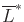, for the sequential game. The security plan is defined by providing the action that selects the lowest cost child vertex, for each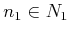. Ifresponds rationally to the security plan of, then the path shown in bold in Figure 10.14d will be followed. The execution of's security plan yields the action sequence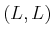forand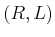for. The upper value is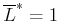.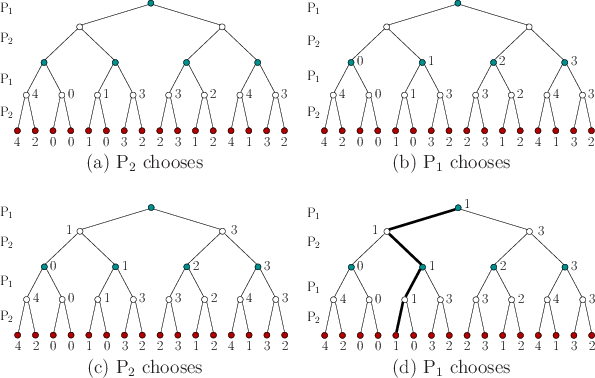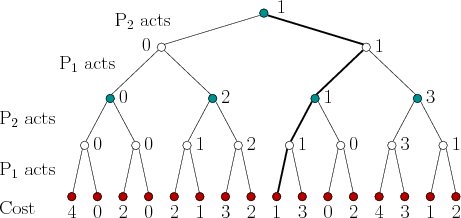A security plan forcan be computed similarly; however, the order of the decisions must be swapped. This is not easy to visualize, unless the order of the players is swapped in the tree. Ifacts first, then the resulting tree is as shown in Figure 10.15. The costs on the leaves appear in different order; however, for the same action sequences chosen byand, the costs obtained at the end of the game are the same as those in Figure 10.14. The resulting lower value for the game is found to be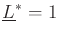. The resulting security plan is defined by assigning the action to each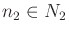that maximizes the cost value of its children. Ifresponds rationally to the security plan of, then the actions executed will beforandfor. Note that these are the same as those obtained from executing the security plan of, even though they appear different in the trees because the player order was swapped. In many cases, however, different action sequences will be obtained.

As in the case of a single-stage game,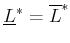implies that the game has a deterministic saddle point and the value of the sequential game is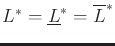. This particular game has a unique, deterministic saddle point. This yields predictable, identical choices for the players, even though they perform separate, worst-case analyses.

A substantial reduction in the cost of computing the security strategies can be obtained by recognizing when certain parts of the tree do not need to be explored because they cannot yield improved costs. This idea is referred to as alpha-beta pruning in AI literature (see , pp. 186-187 for references and a brief history). Suppose that the tree is searched in depth-first order to determine the security strategy for. At some decision vertex for, suppose it has been determined that a cost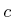would be secured if a particular action,, is applied; however, there are still other actions for which it is not known what costs could be secured. Consider determining the cost that could be secured for one of these remaining actions, denoted by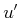. This requires computing howwill maximize cost to respond to. As soon ashas at least one option for which the cost,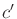, is greater than, the other children ofdo not need to be explored. Why? This is becausewould never chooseifcould respond in a way that leads to a higher cost than whatcan already secure by choosing. Figure 10.16 shows a simple example. This situation can occur at any level in the tree, and when an action does not need to be considered, an entire subtree is eliminated. In other situations, children ofcan be eliminated becausewould not make a choice that allowsto improve the cost below a value thatcan already secure for itself.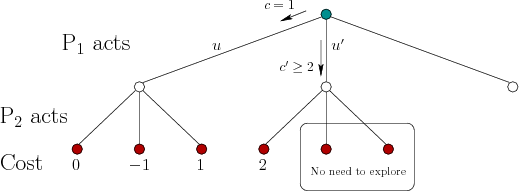Steven M LaValle 2020-08-14